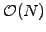SYLLABUS  Previous: 7 WAVELETS  Up: 7 WAVELETS  Next: JBONE user manual

## 7.1 Remain a matter of research

Slide : [ Links ]

There is a growing interest in using wavelets not only for the discretization of functions (sect.1.4), but also to approximate differential and integral operators. Motivations for that are the potential gain of solving global problems with the same numberof operations as there are unknowns, relying on recent advances in iterative methods (sect.3.5) to solve linear systems in sparse format. Having not had the possibility so far to implement wavelets into the JBONE applet and extract the essence of research papers in a pedagogical manner, this section is still limited to a number of links to papers maintained on a web site from MathSoft:

1. D. M. Bond and S. A. Vavasis, Fast Wavelet Transforms for Matrices Arising From Boundary Element Methods.
2. T. Chan, W. Tang and W. Wan, Wavelet sparse approximate inverse preconditioners
3. P. Charton and V. Perrier, Factorisation sur Bases d'Ondelettes du Noyeau de la Chaleur et Algorithmes Matriciels Rapides Associes
4. P. Charton and V. Perrier, Towards a Wavelet Based Numerical Scheme for the Two-Dimensional Navier-Stokes Equations.
5. P. Charton and V. Perrier, A Pseudo-Wavelet Scheme for the Two-Dimensional Navier-Stokes Equations.
6. S. Dahlke and A. Kunoth, Biorthogonal Wavelets and Multigrid.
7. S. Dahlke and I. Weinreich, Wavelet-Galerkin Methods: An Adapted Biorthogonal Wavelet Basis.
8. S. Dahlke and I. Weinreich, Wavelet Bases Adapted to Pseudo-Differential Operators.
9. W. Dahmen and A. Kunoth, Multilevel Preconditioning.
10. R. Glowinski, T. Pan , R. O. Wells, Jr. and X. Zhou, Wavelet and Finite Element Solutions for the Neumann Problem Using Fictitious Domains
11. R. Glowinski, A. Rieder, R. O. Wells, Jr. and X. Zhou, A Wavelet Multigrid Preconditioner for Dirichlet Boundary Value Problems in General Domains.
12. R. Glowinski, A. Rieder, R. O. Wells, Jr. and X. Zhou, A Preconditioned CG-Method for Wavelet-Galerkin Discretizations of Elliptic Problems
13. F. Heurtaux, F. Planchon and M. V. Wickerhauser, Scale Decomposition in Burgers' Equation
14. A. Jiang, Fast wavelet based methods for certain time dependent problems
15. A. Kunoth, Multilevel Preconditioning - Appending Boundary Conditions by Lagrange Multipliers.
16. J. Lewalle, Wavelet Transforms of some Equations of Fluid Mechanics
17. J. Lewalle, Energy Dissipation in the Wavelet-Transformed Navier-Stokes Equations
18. J. Lewalle, On the effect of boundary conditions on the multifractal statistics of incompressible turbulence
19. J. Lewalle, Diffusion is Hamiltonian
20. D. Lu, T. Ohyoshi and L. Zhu, Treatment of Boundary Conditions in the Application of Wavelet-Galerkin Method to a SH Wave Problem
21. A. Rieder and X. Zhou, On the Robustness of the Damped V-Cycle of the Wavelet Frequency Decompositions Multigrid Method
22. A. Rieder, R. O. Wells, Jr. and X. Zhou, A Wavelet Approach to Robust Multilevel Solvers for Anisotropic Elliptic Problems.
23. A. Rieder, R. O. Wells, Jr. and X. Zhou, On the Wavelet Frequency Decomposition Method
24. O. V. Vasilyev and S. Paolucci, A Dynamically Adaptive Multilevel Wavelet Collocation Method for Solving Partial Differential Equations in a Finite Domain.
25. O. V. Vasilyev, S. Paolucci and M. Sen, A Multilevel Wavelet Collocation Method for Solving Partial Differential Equations in a Finite Domain.
26. R. O. Wells, Jr. and X. Zhou, Wavelet Solutions for the Dirichlet Problem
27. R. O. Wells, Jr. and X. Zhou, Wavelet Interpolation and Approximate Solution of Elliptic Partial Differential Equations
28. R. O. Wells, Jr. and X. Zhou, Representing the Geometry of Domains by Wavelets with Applications to Partial Differential Equations
29. R. O. Wells, Jr., Multiscale Applications of Wavelets to Solutions f Partial Differential Equations

SYLLABUS  Previous: 7 WAVELETS  Up: 7 WAVELETS  Next: JBONE user manual